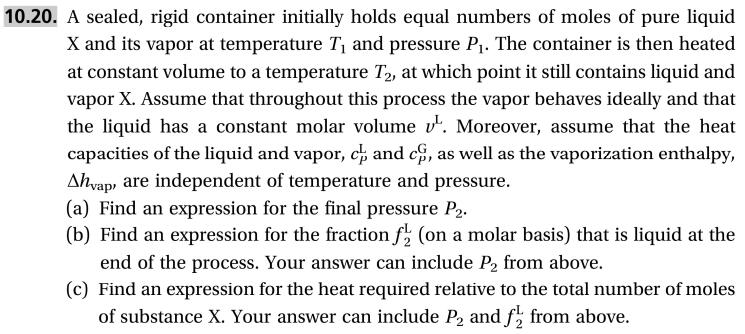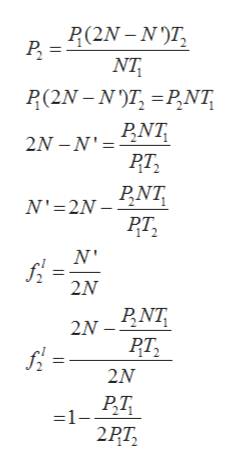# 10.20. A sealed, rigid container initially holds equal numbers of moles of pure liquidX and its vapor at temperature T and pressure P. The container is then heatedat constant volume to a temperature T2, at which point it still contains liquid andvapor X. Assume that throughout this process the vapor behaves ideally and thatthe liquid has a constant molar volume v. Moreover, assume that the heatcapacities of the liquid and vapor, ch and c,Ahyap are independent of temperature and pressure(a) Find an(b) Find an expression for the fraction f (on a molar basis) that is liquid at theend of the process. Your answer can include P2 from above.(c) Find an expression for the heat required relative to the total number of molesof substance X. Your answer can include P and f from above.as well as the vaporization enthalpyexpression for the final pressure P2

Question
37 views

This problem is (10.20) from a book  "Thermodynamics and Statistical Mechanics An Integrated Approach by M. Scott Shell"help_outlineImage Transcriptionclose10.20. A sealed, rigid container initially holds equal numbers of moles of pure liquid X and its vapor at temperature T and pressure P. The container is then heated at constant volume to a temperature T2, at which point it still contains liquid and vapor X. Assume that throughout this process the vapor behaves ideally and that the liquid has a constant molar volume v. Moreover, assume that the heat capacities of the liquid and vapor, ch and c, Ahyap are independent of temperature and pressure (a) Find an (b) Find an expression for the fraction f (on a molar basis) that is liquid at the end of the process. Your answer can include P2 from above. (c) Find an expression for the heat required relative to the total number of moles of substance X. Your answer can include P and f from above. as well as the vaporization enthalpy expression for the final pressure P2 fullscreen
check_circle

Step 1

In the given problem there is a rigid, sealed container containing equal number of moles of liquid X and its vapor is heated from Temperature T1 to T2 that caused some vaporization of liquid into its vapor.

State 1

Pressure in container= P1

Temperature in container= T1

Moles of vapor=moles of liquid = N

State 2

Pressure in container= P2

Temperature in container= T2

Let number of moles remain in container be = N’

Then number of moles of vapor phase = 2N-N’

Step 2

(a) Assuming volume of liquid phase is negligible compared to vapor phase.

Ideal gas equation:

Step 3

(b) From the above expression de...help_outlineImage TranscriptioncloseP(2N-NT ΝΤ P(2N-NTPNT 2N-N' PNΤ PT NΝ'= 2N-DNT PT Ν' 2Ν 2N-Nη 2N -1_β7 2PT ς fullscreen

### Want to see the full answer?

See Solution

#### Want to see this answer and more?

Solutions are written by subject experts who are available 24/7. Questions are typically answered within 1 hour.*

See Solution
*Response times may vary by subject and question.
Tagged in

### Thermodynamics